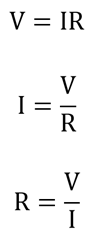# DC (Direct Current) Circuits

It consists of a direct current source with a conducting wire passing from one source terminal to a set of electrical devices and then back to the other terminal, forming a complete circuit. A battery or a DC generator is commonly used as a power source in DC circuits. The wires and also the main element in the device that are used in circuits must be a conductor of electricity. The passing electricity consists of voltage, current and resistance, which are measured in Volts, Amperes and Ohms, respectively.

A simple DC circuit set-up consisting of a battery, bulb and a switch to activate the device is shown in the figure below.

FIGURE

In this, the current flows in a loop from one battery end to the bulb and forms a circuit. The switch in the circuit turns the power on or off as and when needed.

### Ohm’s Law

This law states the relationship between voltage, current and resistance. It can be expressed in any of the following three ways based on the requirement of any of the three stated quantities.V = voltage (V)

I = current (A)

R = resistance (Ω)